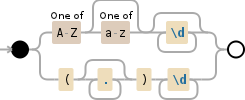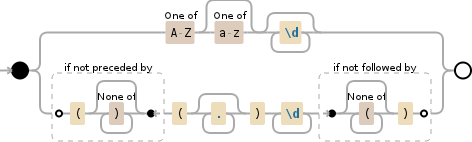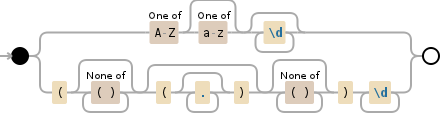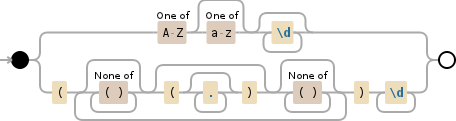# RegEx for parsing chemical formulas

(PO4)2 really sits aside from all.

Let's start from simple, match items without parenthesis:

[A-Z][a-z]?\d*


Using regex above we can successfully parse Ag3PO4, H2O, CH3OOH.

Then we need to somehow add expression for group. Group by itself can be matched using:

$$.*?$$\d+


So we add or condition:

[A-Z][a-z]?\d*|$$.*?$$\d+Demo

Which works for given cases. But may be you have some more samples.

Note: It will have problems with nested parenthesis. Ex. Co3(Fe(CN)6)2

If you want to handle that case, you can use the following regex:

[A-Z][a-z]?\d*|(?<!$$[^)]*)\(.*$$\d+(?![^(]*\))For Objective-C you can use the expression without lookarounds:

[A-Z][a-z]?\d*|$$[^()]*(?:\(.*$$)?[^()]*\)\d+Demo

Or regex with repetitions (I don't know such formulas, but in case if there is anything like A(B(CD)3E(FG)4)5 - multiple parenthesis blocks inside one.

[A-Z][a-z]?\d*|$$(?:[^()]*(?:\(.*$$)?[^()]*)+\)\d+Demo

/($$?)([A-Z])([a-z]*)([0-9]*)($$)?([0-9]*)/g


Play around with it here: http://refiddle.com/

When you encounter a parenthesis group, you don't want to parse what's inside, right?

If there are no nested parenthesis groups you can simply use

[A-Z][a-z]*\d*|$$[^)]+$$\d*


\d is a shorcut for [0-9], [^)] means anything but a parenthesis.

See demo here.